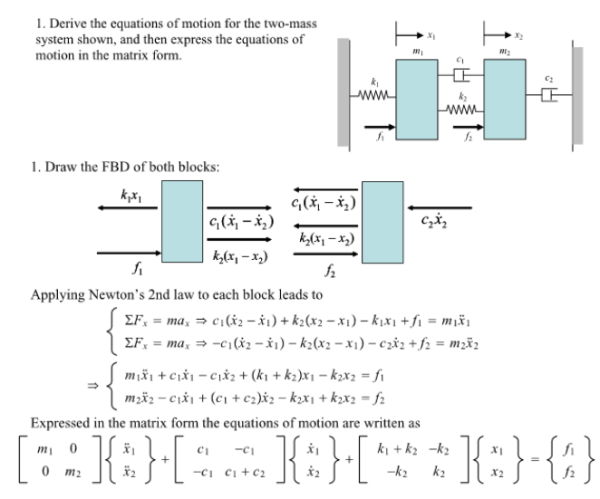Derive the equations of motion for the two-mass system shown, and then express the equations of motion in the matrix form.Derive the equations of motion for the two-mass system shown, and then express the equations of motion in the matrix form.

System Dynamics Page 2 dynamics dynamics dynamics dynamics dynamics dynamics dynamics System dynamics Page 3